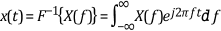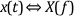# Fourier Transform

## LabVIEW 2018 Help

Edition Date: March 2018
Part Number: 371361R-01
View Product InfoLabVIEW 2015 HelpLabVIEW 2016 HelpLabVIEW 2017 HelpLabVIEW 2018 HelpLabVIEW 2019 Help

The most common applications of the Fourier transform are the analysis of linear time-invariant systems and spectral analysis.

The following equation defines the two-sided Fourier transform.The following equation defines the two-sided inverse Fourier transform.Two-sided means that the mathematical implementation of the forward and inverse Fourier transform considers all negative and positive frequencies and time of the signal. Single-sided means that the mathematical implementation of the transforms considers only the positive frequencies and time history of the signal.

A Fourier transform pair consists of the signal representation in both the time and frequency domain. The following relationship commonly denotes a Fourier transform pair.